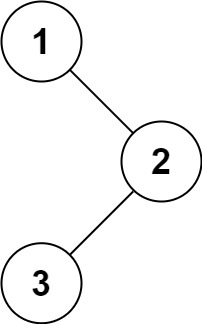# LeetCode - #145 二叉樹的後序遍歷

## 前言

LeetCode 演算法到目前我們已經更新到 144 期，我們會保持更新時間和進度（週一、週三、週五早上 9:00 釋出），每期的內容不多，我們希望大家可以在上班路上閱讀，長久積累會有很大提升。

## 2. 示例```輸入：root = [1,null,2,3] 輸出：[3,2,1]```

```輸入：root = [] 輸出：[]```

```輸入：root =  輸出：```

• 樹中節點數目在範圍 `[0, 100]`
• `-100 <= Node.val <= 100`

## 3. 答案

```swift /* * * Definition for a binary tree node. * public class TreeNode { * public var val: Int * public var left: TreeNode? * public var right: TreeNode? * public init(_ val: Int) { * self.val = val * self.left = nil * self.right = nil * } * } /

class PostorderTraversal { func postorderTraversal(root: TreeNode?) -> [Int] { var res = Int var stack = TreeNode var node = root

``````    while !stack.isEmpty || node != nil {
if node != nil {
res.insert(node!.val, atIndex: 0)
stack.append(node!)
node = node!.right
} else {
node = stack.removeLast().left
}
}

return res
}
``````

} ```

• 主要思想：使用堆疊來幫助迭代樹，向右插入頭部。
• 時間複雜度： O(n)
• 空間複雜度： O(n)